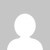# Machine Learning Question With Answers Module 2

[wptelegram-join-channel]

## 17CS73 Machine Learning Question With Answers Module 2

### MODULE 2 – DECISION TREE LEARNING

1. What is decision tree and decision tree learning?

2. Explain representation of decision tree with example.

3. What are appropriate problems for Decision tree learning?

4. Explain the concepts of Entropy and Information gain.

5. Describe the ID3 algorithm for decision tree learning with example

6. Give Decision trees to represent the Boolean Functions:

1. A && ~ B
2. A V [B && C]
3. A XOR B
4. [A&&B] V [C&&D]

7. Give Decision trees for the following set of training examples

8. Consider the following set of training examples.

• What is the entropy of this collection of training examples with respect to the target function classification?
• What is the information gain of a2 relative to these training examples?

9. Identify the entropy, information gain and draw the decision trees for the following set of training examples

10. Construct the decision tree for the following tree using ID3 Algorithm,

11. Discuss Hypothesis Space Search in Decision tree Learning.

12. Discuss Inductive Bias in Decision Tree Learning.

13. What are Restriction Biases and Preference Biases and differentiate between them.

14. Write a note on Occam’s razor and minimum description principal.

15. What are issues in learning decision trees

### Summary:

Her you find the Machine Learning Question With Answers Module 2.

### 2 thoughts on “Machine Learning Question With Answers Module 2”

1.Im not able to find machine learning questions with answers

1.Working on it will be uploaded soon